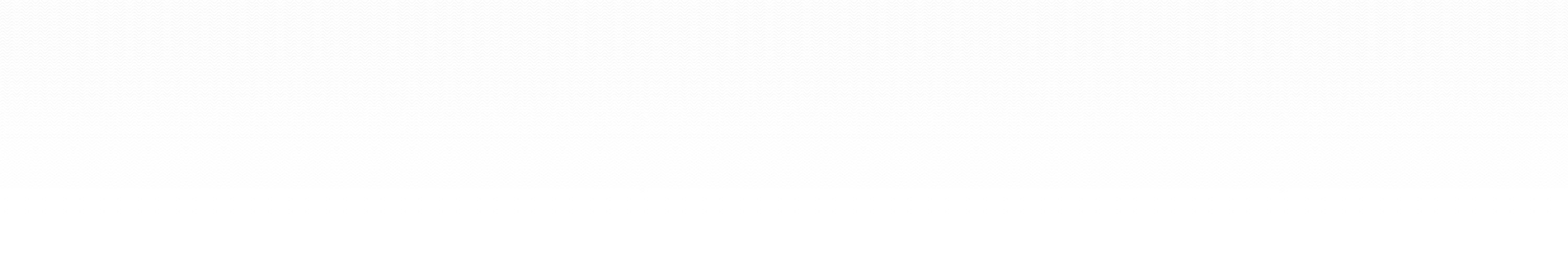### Maple code

Right-click (Windows) or ctrl-click (Mac) to download the files to your machine. You can expect frequent minor modifications to these files, so check back often and think twice before printing.

A worksheet showing the suggested format for assignment submissions [Winter 2012]
A basic introduction to the syntax and features of MAPLE [pdf | Summer 2015]
A quick tutorial on Maple's dsolve/numeric command to find numerical solutions to ordinary differential equations [pdf | Winter 2012]
Derivation of the simpliest stencils for a class of first order ODEs [pdf | Winter 2012]
Introduction of Runge-Kutta stencils in general, and the Huen (modified Euler) and classic fourth order method in particular [pdf | Winter 2012]
Derivation of the Huen method
Definition of absolute stability for one-step stencils with numerical examples of stable and unstable simulations. Derivation and plots of stability regions in the complex plane. Discussion of the stability of numerical solutions to nonlinear problems. [pdf | Winter 2012]
A brief introduction to partial differential equations and Maple's pdsolve command [pdf | Winter 2012]
Derivation of one-sided and centered stencils for higher order derivatives and their application in the forward-time center-space (FTCS), method of lines, and Crank-Nicholson numeric solution of the diffusion equation. Also includes an discussion of the stability analysis of the stencils, and an introduction to the von Neumann stability analysis. [pdf | Winter 2012]
Derivation of higher-derivative stencils, how to code FTCS and Crank-Nicholson for the diffusion equation.
Derivation and stability analysis of several stencils for the advection equation, and implementation of numeric solutions with periodic boundary conditions [pdf | Winter 2012]
Solving the diffusion equation with a nonlinear potential using forward-time centered-space and Crank-Nicholson stencils; also, examples of code to solve nonlinear algebraic systems of equations using Newton's method [pdf | Winter 2012]
How to solve boundary value problems for second order ODEs using Maple's dsolve, as well as shooting, matrix, Newton, and relaxation methods (under construction) [pdf | Winter 2012]
Solution of the Laplace and Poisson equations in 2D using five-point and nine-point stencils for the Laplacian [pdf | Winter 2012]
Discussion of the finite element method in one spatial dimension for elliptic boundary value problems, as well as parabolic and hyperbolic initial value problems. (We assume piecewise linear elements.) [pdf | Winter 2012]
Discussion of the finite element method in two spatial dimensions for elliptic boundary value problems, as well as parabolic and hyperbolic initial value problems. (We assume piecewise linear elements.) [pdf | Winter 2012]